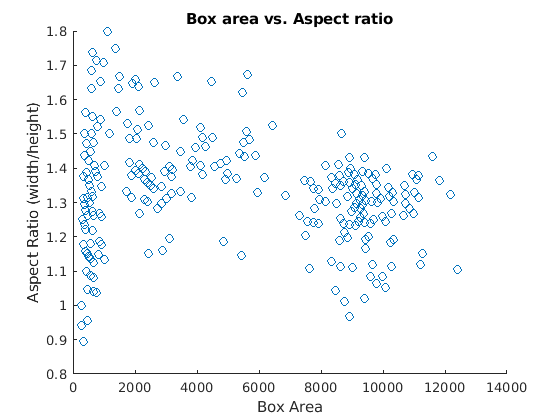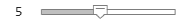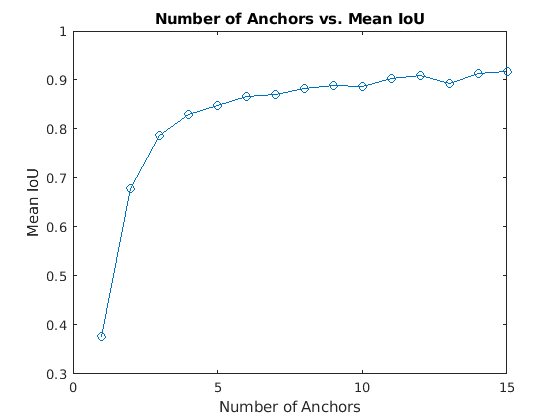# estimateAnchorBoxes

Estimate anchor boxes for deep learning object detectors

## Syntax

``anchorBoxes = estimateAnchorBoxes(trainingData,numAnchors)``
``[anchorBoxes,meanIoU] = estimateAnchorBoxes(trainingData,numAnchors)``

## Description

example

````anchorBoxes = estimateAnchorBoxes(trainingData,numAnchors)` estimates the specified number of anchor boxes using the training data.```
````[anchorBoxes,meanIoU] = estimateAnchorBoxes(trainingData,numAnchors)` additionally returns the mean intersection-over-union (IoU) value of the anchor boxes in each cluster.```

## Examples

collapse all

This example shows how to estimate anchor boxes using a table containing the training data. The first column contains the training images and the remaining columns contain the labeled bounding boxes.

```data = load('vehicleTrainingData.mat'); trainingData = data.vehicleTrainingData;```

Create a `boxLabelDatastore` object using the labeled bounding boxes from the training data.

`blds = boxLabelDatastore(trainingData(:,2:end));`

Estimate the anchor boxes using the `boxLabelDatastore` object.

```numAnchors = 5; anchorBoxes = estimateAnchorBoxes(blds,numAnchors);```

Specify the image size.

`inputImageSize = [128,228,3];`

Specify the number of classes to detect.

`numClasses = 1;`

Use a pretrained ResNet-50 network as a base network for the YOLO v2 network.

`network = resnet50();`

Specify the network layer to use for feature extraction. You can use the `analyzeNetwork` function to see all the layer names in a network.

`featureLayer = 'activation_49_relu';`

Create the YOLO v2 object detection network.

`lgraph = yolov2Layers(inputImageSize,numClasses,anchorBoxes,network, featureLayer)`
```lgraph = LayerGraph with properties: Layers: [182×1 nnet.cnn.layer.Layer] Connections: [197×2 table] InputNames: {'input_1'} OutputNames: {'yolov2OutputLayer'} ```

Visualize the network using the network analyzer.

`analyzeNetwork(lgraph)`

Anchor boxes are important parameters of deep learning object detectors such as Faster R-CNN and YOLO v2. The shape, scale, and number of anchor boxes impact the efficiency and accuracy of the detectors.

For more information, see Anchor Boxes for Object Detection.

Load the vehicle dataset, which contains 295 images and associated box labels.

```data = load('vehicleTrainingData.mat'); vehicleDataset = data.vehicleTrainingData; ```

Add the full path to the local vehicle data folder.

```dataDir = fullfile(toolboxdir('vision'),'visiondata'); vehicleDataset.imageFilename = fullfile(dataDir,vehicleDataset.imageFilename);```

Display the data set summary.

`summary(vehicleDataset)`
```Variables: imageFilename: 295×1 cell array of character vectors vehicle: 295×1 cell ```

Visualize Ground Truth Box Distribution

Visualize the labeled boxes to better understand the range of object sizes present in the data set.

Combine all the ground truth boxes into one array.

`allBoxes = vertcat(vehicleDataset.vehicle{:});`

Plot the box area versus the box aspect ratio.

```aspectRatio = allBoxes(:,3) ./ allBoxes(:,4); area = prod(allBoxes(:,3:4),2); figure scatter(area,aspectRatio) xlabel("Box Area") ylabel("Aspect Ratio (width/height)"); title("Box Area vs. Aspect Ratio")```The plot shows a few groups of objects that are of similar size and shape, However, because the groups are spread out, manually choosing anchor boxes is difficult. A better way to estimate anchor boxes is to use a clustering algorithm that can group similar boxes together using a meaningful metric.

Estimate Anchor Boxes

Estimate anchor boxes from training data using the `estimateAnchorBoxes` function, which uses the intersection-over-union (IoU) distance metric.

A distance metric based on IoU is invariant to the size of boxes, unlike the Euclidean distance metric, which produces larger errors as the box sizes increase . In addition, using an IoU distance metric leads to boxes of similar aspect ratios and sizes being clustered together, which results in anchor box estimates that fit the data.

Create a `boxLabelDatastore` using the ground truth boxes in the vehicle data set. If the preprocessing step for training an object detector involves resizing of the images, use `transform` and `bboxresize` to resize the bounding boxes in the `boxLabelDatastore` before estimating the anchor boxes.

`trainingData = boxLabelDatastore(vehicleDataset(:,2:end));`

Select the number of anchors and estimate the anchor boxes using `estimateAnchorBoxes` function.

```numAnchors =5; [anchorBoxes,meanIoU] = estimateAnchorBoxes(trainingData,numAnchors); anchorBoxes```
```anchorBoxes = 5×2 21 27 87 116 67 92 43 61 86 105 ```

Choosing the number of anchors is another training hyperparameter that requires careful selection using empirical analysis. One quality measure for judging the estimated anchor boxes is the mean IoU of the boxes in each cluster. The `estimateAnchorBoxes` function uses a k-means clustering algorithm with the IoU distance metric to calculate the overlap using the equation, `1 - ``bboxOverlapRatio``(allBoxes,boxInCluster)`.

`meanIoU`
```meanIoU = 0.8411 ```

The mean IoU value greater than 0.5 ensures that the anchor boxes overlap well with the boxes in the training data. Increasing the number of anchors can improve the mean IoU measure. However, using more anchor boxes in an object detector can also increase the computation cost and lead to overfitting, which results in poor detector performance.

Sweep over a range of values and plot the mean IoU versus number of anchor boxes to measure the trade-off between number of anchors and mean IoU.

```maxNumAnchors = 15; meanIoU = zeros([maxNumAnchors,1]); anchorBoxes = cell(maxNumAnchors, 1); for k = 1:maxNumAnchors % Estimate anchors and mean IoU. [anchorBoxes{k},meanIoU(k)] = estimateAnchorBoxes(trainingData,k); end figure plot(1:maxNumAnchors,meanIoU,'-o') ylabel("Mean IoU") xlabel("Number of Anchors") title("Number of Anchors vs. Mean IoU")```Using two anchor boxes results in a mean IoU value greater than 0.65, and using more than 7 anchor boxes yields only marginal improvement in mean IoU value. Given these results, the next step is to train and evaluate multiple object detectors using values between 2 and 6. This empirical analysis helps determine the number of anchor boxes required to satisfy application performance requirements, such as detection speed, or accuracy.

## Input Arguments

collapse all

Training data, specified as a datastore that returns a cell array or table with two or more columns. The bounding boxes must be in a cell array of M-by-4 matrices in the format [x,y,width,height].

The datastore must be one of the following:

Number of anchor boxes for the function to return, specified as an integer.

## Output Arguments

collapse all

Anchor boxes, returned as an N-by-2 matrix, where N is the number of anchor boxes and each entry has the format [height, width]. Use `numAnchors` to specify the number of anchor boxes.

Distance metric, returned as a scalar value. The distance metric provides the mean intersection-over-union (IoU) value of the anchor boxes in each cluster. To ensure anchor boxes overlap well with the boxes in the training data, the `meanIoU` value must be greater than 0.5. The k-means clustering algorithm uses the IoU distance metric to calculate the overlap using the equation `1`-`bboxOverlapRatio`(`box1,box2`).

## Version History

Introduced in R2019b